# AP Chemistry : Molecular Formula

## Example Questions

← Previous 1

### Example Question #1 : Molecular Formula

A neutral ionic compound consisting of a calcium ionic species and a hydroxide ionic species can be predicted to have which of the following molecular formulas?

Ca2(OH)3

Ca(OH)2

Ca(OH)3

Ca2OH

CaOH

Ca(OH)2

Explanation:

Here we are looking for the molecular formula of an ionic compound consisting of Ca and OH. We know that the molecule is neutral, so it must have a charge of 0.  This question is really addressing one's knowledge of typical ionic species.  Calcium ions are found only as Ca2+ and hydroxide ions (OH) are found only as OH–.  Thus for these two species to exist in an neutral ionic compound the only possible formula is Ca(OH)2.

### Example Question #1 : Molecules

What is the correct formula for a compound made of magnesium and nitrogen?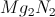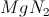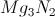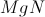Explanation:

To find the correct formula, the charge contributions from each compound must cancel out to zero. Each magnesium ion has a charge of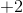and each nitrogen ion has a charge of, due to their respective valence electron configurations. Three magnesium atoms would produce a charge of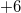, and two nitrogen atoms would produce a charge of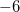. This would thus create a neutral, stable compound.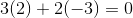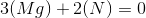### Example Question #2 : Molecular Formula

What is the proper empirical formula for a compound composed of lithium and oxygen?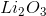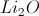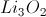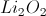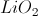Explanation:

To find the correct formula, the charge contributions from each compound must cancel out to zero. Each lithium ion has a charge of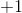and each oxygen ion has a charge of, due to their respective valence electron configurations. Two lithium atoms would produce a charge of, and one oxygen atom would produce a charge of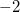. This would thus create a neutral, stable compound.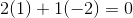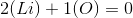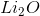### Example Question #4 : Molecules

Which of the following compounds has a percent composition of carbon, (approximately 63%)?

Acetone

Glucose

Butanol

C3H8

Acetone

Explanation:

Acetone, which as a formula of C3H6O has a total molecular weight of: 3(12) + 6(1) + 1(16) = 58g/mole, and the percent of this that carbon makes up is (3(12)/58) X100 = 63%.

### Example Question #1 : Molecules

What is the formula for the ionic compound iron(III) oxide?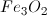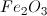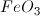Explanation:

When finding the formula for a neutral compound, you must make sure that the positive charges on the cation are cancelled out by the negative charges on the anion. Iron is a transitional element, and can create a couple of different cations depending on how many electrons it loses. The roman numeral III tells you the charge on the iron cation is 3+ (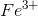). Oxygen as an anion carries a charge of -2 (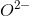).  To make the compound neutral, the charges from oxygen must equal the charges from iron.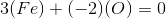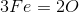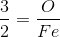This solution tells us the ratio of oxygen to iron: for every three oxygen, there are two iron.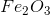gives a total cation charge of +6 (2 iron ions), and a total anion charge of -6 (3 oxygen anions). These charges offset each other, resulting in a neutral compound.

### Example Question #33 : Ap Chemistry

Which compound has both ionic and covalent bonds?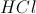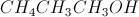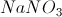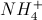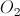Explanation:

In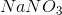, the nitrogen is bound covalently to the three oxygens, and the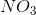complex has an overall of.

Sodium has a charge of, and is ionically bound to thecomplex.

When put into water, the compound will dissociate into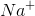and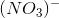.

HCl is an ionic compound, while the other answer choices have only covalent bonds.

### Example Question #7 : Molecules

Which of the following compounds breaks the octet rule?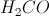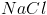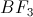Explanation:

The octet rule requires that each atom in the molecule has eight valence electrons, fully filling the s and p subshells. Atoms that have a full octet tend to be more stable and lower in energy.

In the compound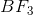, each fluorine has seven initial valence electrons and boron has three initial valence electrons. Upon forming the compound bonds, boron shares its three electrons with each fluorine through covalent bonds. This givens each fluorine a full octet, but leave boron with only three covalent bonds, resulting in only six valence electrons. Boron does not satisfy the octet rule in this compound.

Each atom in the other listed compounds forms bonds to generate a full octet. Sodium chloride achieves this through an ionic bond. Methanal and diatomic oxygen both use double bonds to help achieve octets.

### Example Question #33 : Compounds And Molecules

The molecular formula of a compound __________.

is usually a multiple of the empirical formula

is not related to the empirical formula

is impossible to determine

is constant regardless of the compound

is usually a multiple of the empirical formula

Explanation:

The empirical formula of a compound is the simplest whole number ratio of the elements in the compound, whereas the molecular formula gives the exact number of atoms of each element in the compound—this is usually a multiple of the empirical formula. If the empirical formula of a compound is CH, a common molecular formula is C6H6 (benzene).

### Example Question #3 : Molecules

What is the molecular formula for Cobalt (II) Nitrate?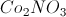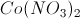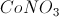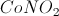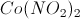Explanation:

Cobalt (II) is Cobalt in its second oxidation state,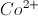, and the chemical formula for Nitrate is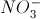. The neutral salt is.

### Example Question #10 : Molecules

What is the molecular formula for ferrous sulfide?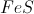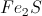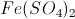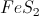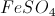Ferrous is the name for Iron (II), or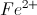. Sulfide is the anion of sulfur,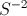. The chemical formula for ferrous sulfide is.